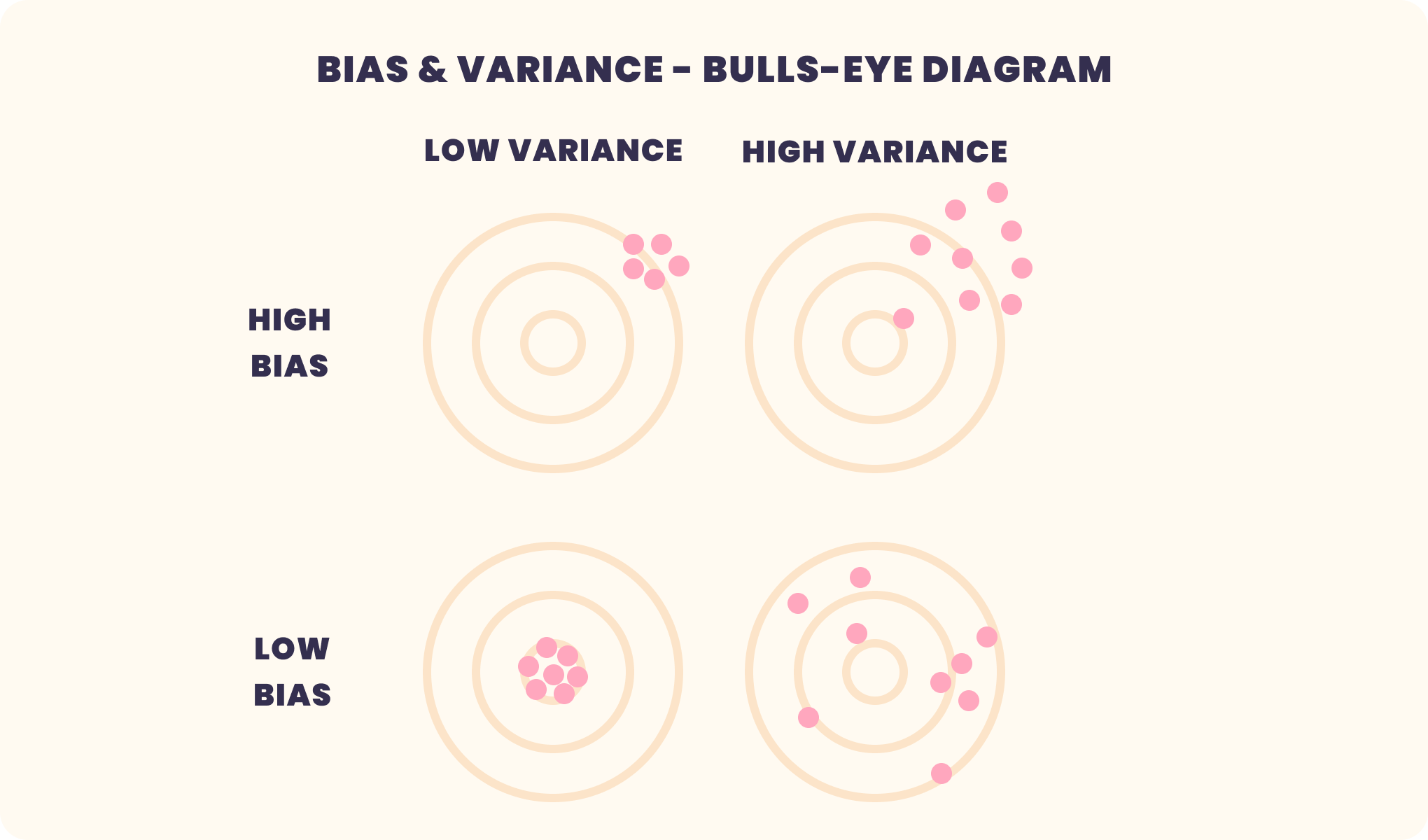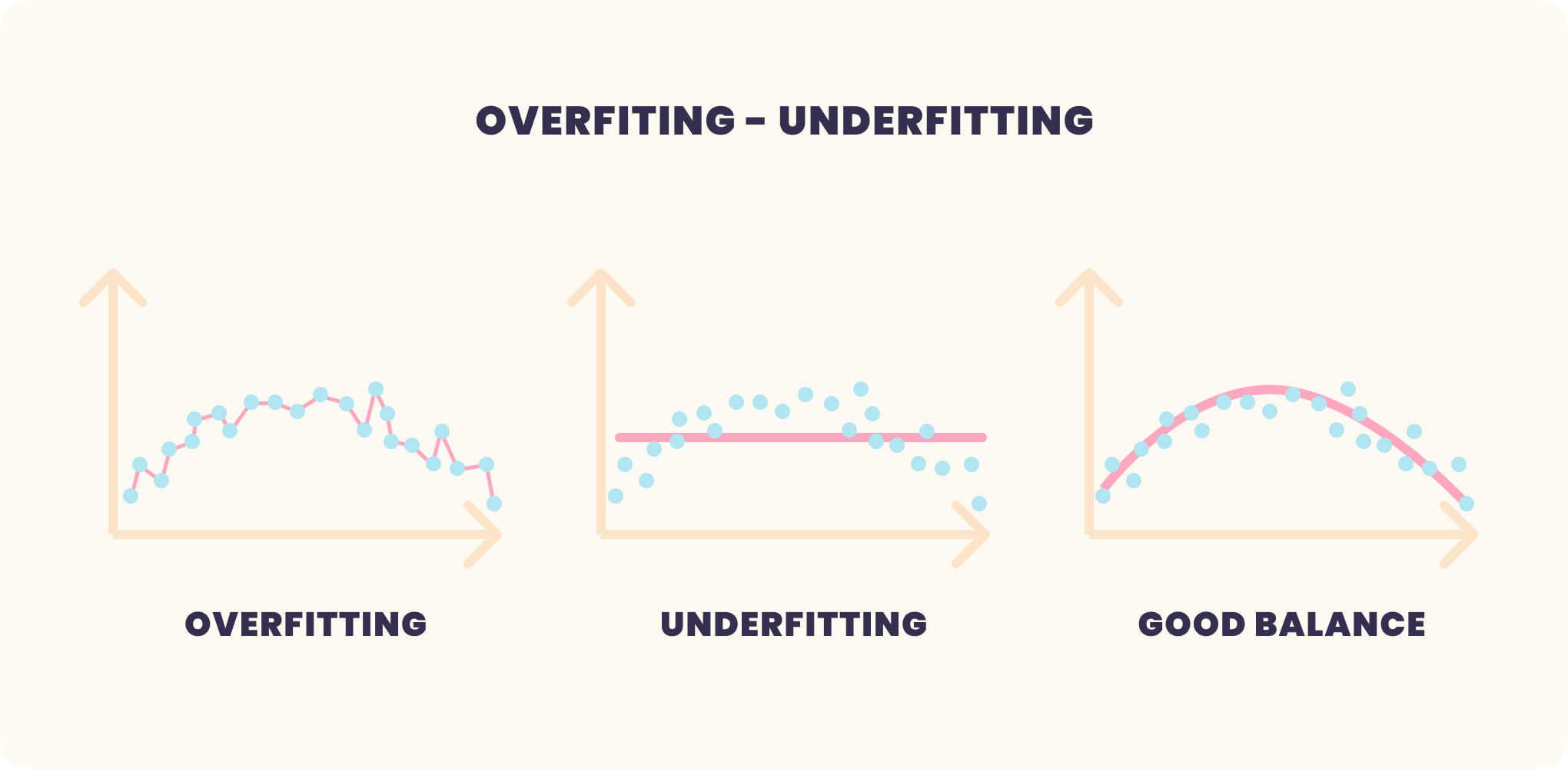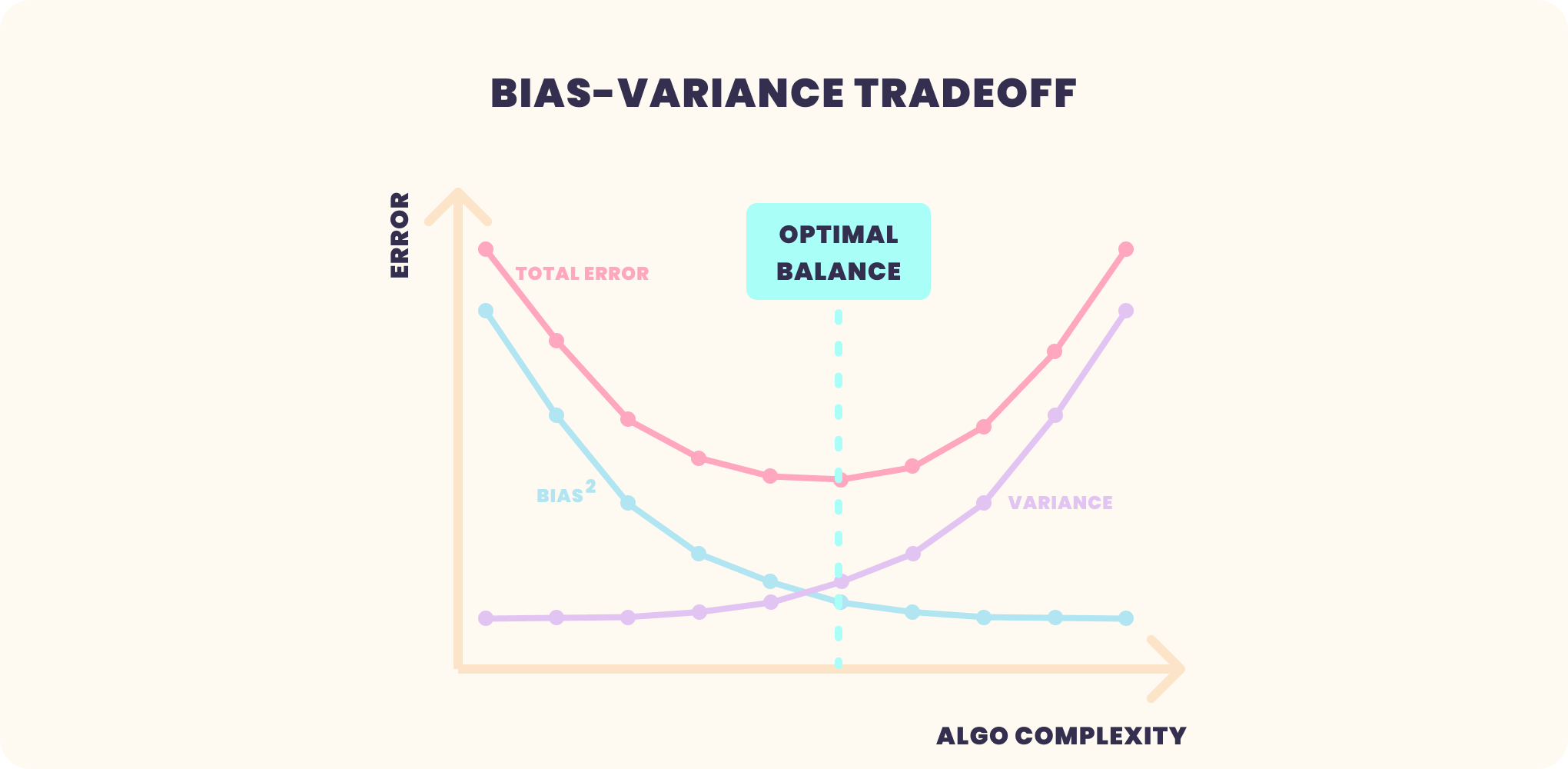# Trade-off between bias and variance

PUBLISHED
2021.02.17 (Wed)
CATEGORY
Machine Learning Beginner
TOPIC
Machine Learning Model Performance
12分31秒

In machine learning, if you want to improve the prediction performance of your model, it is important to understand the parameters related to prediction error and accuracy.
In the first place, the purpose of machine learning is to predict unknown data.
And if you want to create a model with good prediction performance, you need to understand what is described in this article.
So, in this article, I will explain the relationship between variance, bias, and noise and the predictive performance of a model.

## Bias-Variance Decomposition

To improve the prediction accuracy of a model, we need to minimize the generalization error.
The generalization error can be divided into the following three categories

• Bias (Bias)
• Variance
• Noise (Irreducible Error)

Dividing the elements in this way is called Bias-Variance Decomposition.
Since this is an introductory article and there may be differences in literacy in reading formulas, I will not describe the actual formulas here, but the relationship between generalization performance and bias, variance, and noise is as followsNow, let's take a closer look at the decomposed elements.

Bias: Bias
Bias in machine learning (and statistics) is the difference between the average predicted value of a model and the true value it is trying to predict.
A model with a high bias is in a state of under-learning, where the relationship between the inputs to the model and the outputs has not been accurately learned, and even training data cannot be accurately predicted.
AI & Machine Learning Knowledge Center: Bias

Variance: Variance
Variances in machine learning (and statistics) are values that indicate the spread of model predictions.
AI & Machine Learning Knowledge Center: Variance

Noise: Irreducible Error
An unreducible error, a measure of the amount of noise in the data.
It is important to understand that no matter how good your model is, there will always be noise in your data that cannot be removed.

Generalization Error
This is the error between the true value and the prediction of unknown data.
The goal of machine learning is to predict unknown data, so the generalization error is used to evaluate whether it was able to predict the unknown data.

## Underfitting and overfitting

The figure below shows the relationship between bias and variance like a dart target.
It is often used to explain bias and variance, so you may have seen it before.In this figure, the center of the target represents a model that perfectly predicts the correct value.
As we move away from the central target, the predictions get worse and worse.

Let's take a look at each of these states.

### High bias, low variance (top left)

This state is called underfitting and occurs when the trained model does not fully capture the patterns in the data.
This situation occurs when the amount of data to build the model is very small, or when a linear algorithm is applied to non-linear data to build a linear model.
In this case, the learned model is very simple.

### Low bias, high variance (bottom right)

This state is called overfitting, and it occurs when the learned model learns patterns in the data, but also learns noise. Overtraining on a noisy dataset can lead to this situation.
In this case, the learned model is in a complex state.

The figure below is a good reference for overfitting and underfitting.## Trade-off between bias and variance

The figure below shows the relationship between bias, variance, and generalization error, with model complexity on the horizontal axis and error (Error) on the vertical axis.On the horizontal axis, the further to the left you go, the higher the bias and the lower the variance.
In this case, we can see that the generalization error is very high and the prediction performance of the model is not good.

On the other hand, the further to the right you go, the lower the bias and the higher the variance.
As you move to the right, the bias becomes lower and the variance becomes higher, the generalization error becomes much larger, and the prediction performance of the model is not good as well.
To get a good prediction performance of the model, we need to find the bias and variance of the light blue line in the figure where the generalization error is minimized.
Therefore, understanding the bias and variance is very important to understand the behavior of the prediction model.

## Optimizing the generalization error

There are various approaches to optimize the generalization error, an example is given below.

VARISTAは機械学習モデルの開発、管理をノーコードで効率的に行うことができる新しいプラットフォームです。
データをお持ちでしたらすぐに始められますので、是非ともご相談ください。
Stay in Touch